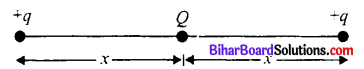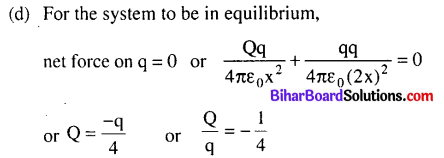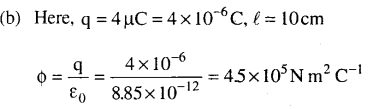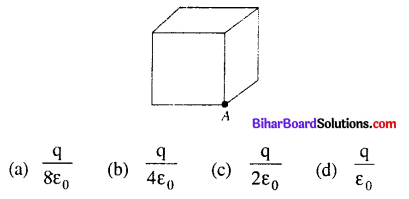Bihar Board 12th Physics Objective Questions and Answers

## Bihar Board 12th Physics Objective Answers Chapter 1 Electric Charges and Fields

Physics 12th Chapter 1 Objective Bihar Board Question 1.
Object may acquire an excess or deficiency of charge by
(a) electric force
(b) heating
(c) shaking
(d) by rubbing
(d) by rubbing

Class 12 Physics Chapter 1 Objective Questions Bihar Board Question 2.
The charge on an electron was calculated by
(b) J.J. Thomson
(c) Millikan
(d) Einstein
(c) Millikan

Electrostatics Class 12 Notes Bihar Board Question 3.
A method for charging a conductor without bringing a charged body in contact with it is called
(a) Magnetization
(b) Electrification
(c) Electrostatic induction
(d) Electromagnetic induction
(c) Electrostatic induction

Physics Class 12 Chapter 1 Objective Questions Bihar Board Question 4.
The number of electrons present in -1 C of charge is
(a) 6 × 1018
(b) 1.6 × 1019
(c) 6 × 1019
(d) 1.6 × 1018
(a) 6 × 1018

Electric Charge And Field Objective Question Bihar Board Question 5.
A cup contains 250 g of water. Find the total positive charges present in the cup of water.
(a) 1.34 × 1019 C
(b) 1.34 × 107 C
(c) 2.43 × 1019 C
(d) 2.43 × 107 C
(d) 2.43 × 107 C
Solution:
Mass of water = 250 g,
Molecular mass of water = 18 g
Number of molecules in 18 g of water = 6.02 × 1023
Number of molecules in one cup of water
= $$\frac{250}{18} \times 6.02 \times 10^{23}$$
Each molecule of water contains two hydrogen atoms and one oxygen atom, i.e., 10 electrons and 10 protons.
∴ Total positive charge present in one cup of water
= $$\frac{250}{18}$$ x 6.02 x 1023 × 10 × 1.6 × 10-19C
= 1.34 × 107C

Electric Charges And Fields Class 12 Objective Questions Bihar Board Question 6.
The constant k in Coulomb’s law depends on
(a) nature of medium
(b) system of units
(c) intensity of charge
(d) both (a) and (b)
(a) nature of medium

12th Physics Objective Bihar Board Question 7.
Which of the following statement is not a similarity between electrostatic and gravitational forces ?
(a) Both forces obey inverse square law.
(b) Both forces operate over very large distances.
(c) Both forces are conservative in nature.
(d) Both forces are attractive in nature always.
(d) Both forces are attractive in nature always.

Questions On Electric Charges And Fields Bihar Board Question 8.
SI unit of permittivity of free space is
(b) Weber
(c) C2 N-1m-2
(d) C2 N-1m-1
(c) C2 N-1m-2

Physics Class 12 Chapter 1 Bihar Board Question 9.
The force between two small charged spheres having charges of 1 × 10-7 C and 2 × 10-7 C placed 20 cm apart in air is
(a) 4.5 × 10-2 N
(b) 4.5 × 10-3 N
(c) 5.4 × 10-2 N
(d) 5.4 × 10-3 N
(b) 4.5 × 10-3 N
Solution:Electric Charge And Field Notes In Hindi Bihar Board Question 10.
The ratio of magnitude of electrostatic force and gravitational force for an electron and a proton is
(a) 6.6 × 1039
(b) 2.4 × 1039
(c) 6.6 × 1029
(d) 2.4 × 1029
(b) 2.4 × 1039

Class 12 Physics Chapter 1 Bihar Board Question 11.
Consider the charges q, +q and -q placed at the vertices of an equilateral triangle of each side l. The sum of forces acting on each charge is$$\frac{\mathrm{q}^{2}}{4 \sqrt{2} \pi \varepsilon_{0} \ell^{2}}$$

Class 12 Physics Chapter 1 Electric Charges And Fields Notes Bihar Board Question 12.
A charge Q is placed at the centre of the line joining two point charges +q and +q as shown in Figure. The ratio of charges Q and q is(a) 4
(b) 1/4
(c) -4
(d) -1/4
(d) -1/4
Solution:Electric Charges And Fields Class 12 Bihar Board Question 13.
The force per unit charge is known as
(a) electric flux
(b) electric field
(c) electric potential
(d) electric current
(b) electric field

Physics Objective Question Bihar Board Question 14.
The dimensional formula of electric intensity is
(a) [M1L1T3A-1]
(b) [M1L1T-3A-1]
(c) [M1L2T-3A-1]
(d) [M1L2T1A1]
(c) [M1L2T-3A-1]

Physics Class 12 Chapter 1 Questions And Answers Bihar Board Question 15.
If the charge on an object is doubled then electric field becomes
(a) half
(b) double
(c) unchanged
(d) thrice
(b) double

Electric Charges And Fields Class 12 Important Questions Bihar Board Question 16.
A force of 2.25 N acts on a charge of 15 × 10-4 C. The intensity of electric field at that point is
(a) 150NC-1
(b) 15NC-1
(c) 1500 NC-1
(d) 1.5NC-1
(c) 1500 NC-1

Electric Field Class 12 Bihar Board Question 17.
The electric field that can balance a charged particle of mass 3.2 × 10-27 kg is (Given that the charge on the particle is 1.6 × 10-19 C)
(a) 19.6 × 10-8 N C-1
(b) 20 × 10-6 N C-1
(c) 19.6 × 108 N C-1
(d) 20 × 106 N C-1
(a) 19.6 × 10-8 N C-1
Solution:
(a) Here, m = 3.2 × 10-27 kg, e = 1.6 × 10-19 C, g = 9.8 m s-2Question 18.
Electric field lines provide information about
(a) field strength
(b) direction
(c) nature of charge
(d) all of these
(d) all of these

Question 19.
A non-uniform electric field is represented by the diagram. At which of the following points the electric field is greatest in magnitude ?(a) A
(b) B
(c) C
(d) D
(d) D

Question 20.
Which of the following statements is not true about electric field lines ?
(a) Electric field lines start from positive charge and end at negative charge.
(b) Two electric field lines can never cross each other.
(c) Electrostatic field lines do not form any closed loops.
(d) Electric field lines cannot be taken as continuous curve.
(d) Electric field lines cannot be taken as continuous curve.

Question 21.
The SI unit of electric flux is
(a) N C-1 m2
(b) N C m-2
(c) N C-2 m2
(d) N C-1 m-2
(a) N C-1m2

Question 22.
The dimensional formula of electric flux is
(a) [M1L1T-2]
(b) [M1L3T-3A-1]
(c) [M2L2T-2A-2]
(d) [M1L-3T3A1]
(b) [M1L3T-3A-1]

Question 23.
The unit of electric dipole moment is
(a) newton
(b) coulomb
(d) debye
(d) debye

Question 24.
The surface considered for Gauss’s law is called
(a) Closed surface
(b) Spherical surface
(c) Gaussian surface
(d) Plane surface
(c) Gaussian surface

Question 25.
A sphere encloses an electric dipole within it. The total flux across the sphere is
(a) zero
(b) half that due to a single charge
(c) double that due to a single charge
(d) dependent on the position of dipole.
(a) zero

Question 26.
A point charge 4 µC is at the centre of a cubic Gaussian surface 10 cm on edge. Net electric flux through the surface is
(a) 2.5 × 105 N m2 C-1
(b) 4.5 × 105 N m2 C-1
(c) 4.5 × 106 N m2 C-1
(d) 2.5 × 106 N m2 C-1
(b) 4.5 × 105 N m2 C-1
Solution:Question 27.
The total flux through the faces of the cube with side of length a if a charge q is placed at corner A of the cube is(b) $$\frac{\mathrm{q}}{8 \varepsilon_{0}}$$
Solution (a) In the figure, when a charge q is placed at comer A of the cube, it is being shared equally by 8 cubes.
∴The total flux through the faces of the given cube = $$\frac{q}{8 \varepsilon_{0}}$$

Question 28.
Two infinite plane parallel sheets, separated by a distance d have equal and opposite uniform charge densities σ. Electric field at a point between the sheets is
(a) $$\frac{\sigma}{2 \varepsilon_{0}}$$
(b) $$\frac{\sigma}{\varepsilon_{0}}$$
(c) zero
(d) depends on the location of the point
(b) $$\frac{\sigma}{\varepsilon_{0}}$$

Question 29.
Figure shows electric field lines in which an electric dipole $$\vec{D}$$ is placed as shown. Which of the following : statements is correct?(a) The dipole will not experience any force.
(b) The dipole will experience a force towards right.
(c) The dipole will experience a force towards left.
(d) The dipole will experience a force upwards.